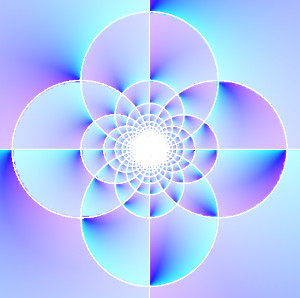# Gauss map, Euclidean algorithm, and continued fractionsThe Gauss map  is the functionwhere ⌊y⌋ is the floor of y, the greatest integer no larger than y.

I’ve written about this map a couple times before. First, I wrote about how this map is measure-preserving. Second, I wrote about the image at the top of the post, based on Michael Trott’s idea of extending the floor function to the complex plane and plotting it.

This post is a third take on the Gauss map, expanding on a comment by Giovanni Panti. His paper opens by saying

The fact that the euclidean algorithm eventually terminates is pervasive in mathematics. In the language of continued fractions, it can be stated by saying that the orbits of rational points under the Gauss map xx−1 −⌊x−1⌋ eventually reach zero.

What does the Gauss map have to do with continued fractions or the Euclidean algorithm? We’ll show this by working through an example.

A continued fraction has the formLet’s start with 162/47 and see how we would write it as a continued fraction. An obvious place to start would be to write this as a proper fraction.Next we turn 21/47 into 1 over something.Now let’s do the same thing with 47/21: turn it into a proper fraction 2 + 5/21, then rewrite the fraction part 5/21 as the reciprocal of its reciprocal:Finally, we write 21/5 as 4 + 1/5, and we’re done:Now go back and look at what happens to the fraction in the bottom left corner at each step:The sequence of bottom left fractions is 21/47, 5/21, 1/5. Each fraction is replaced by its Gauss map: f(21/47) = 5/21, and f(5/21) = 1/5. We applied the Gauss map above naturally in the process of creating a continued fraction.

Now suppose we wanted to find the greatest common divisor of 162 and 47 using the Euclidean algorithm.Notice that these are the same numbers, produced by the same calculations as above.

 There are other things called the Gauss map, such as the map that takes a point on a surface to the unit normal at that point. That’s not the Gauss map we’re talking about here.